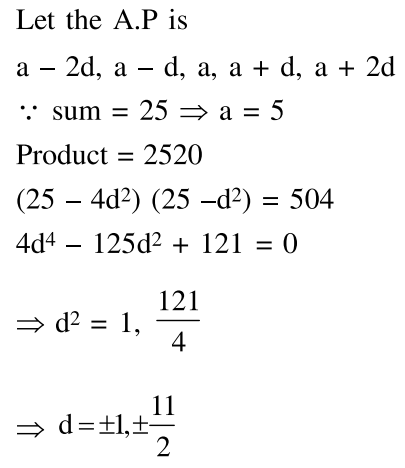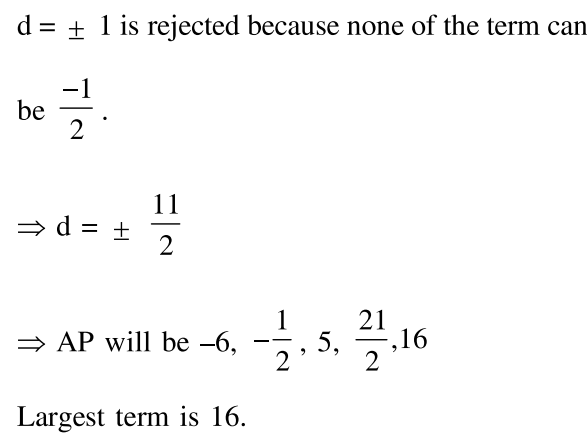# Five numbers are in A.P., whose sum is 25 and product is 2520 .Question:

Five numbers are in A.P., whose sum is 25 and product is 2520 . If one of these five numbers is $-\frac{1}{2}$, then the grea est number amongst them is :

1. $\frac{21}{2}$

2. 27

3. 16

4. 7

Correct Option: , 3

Solution: